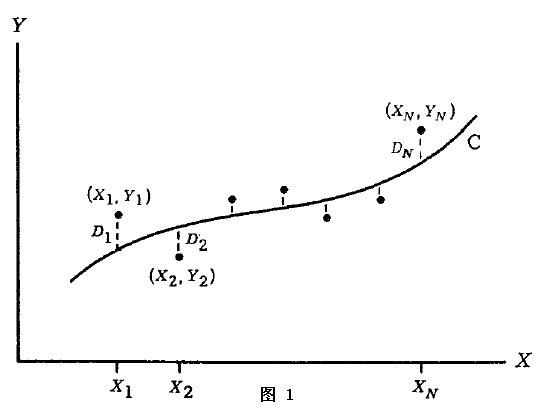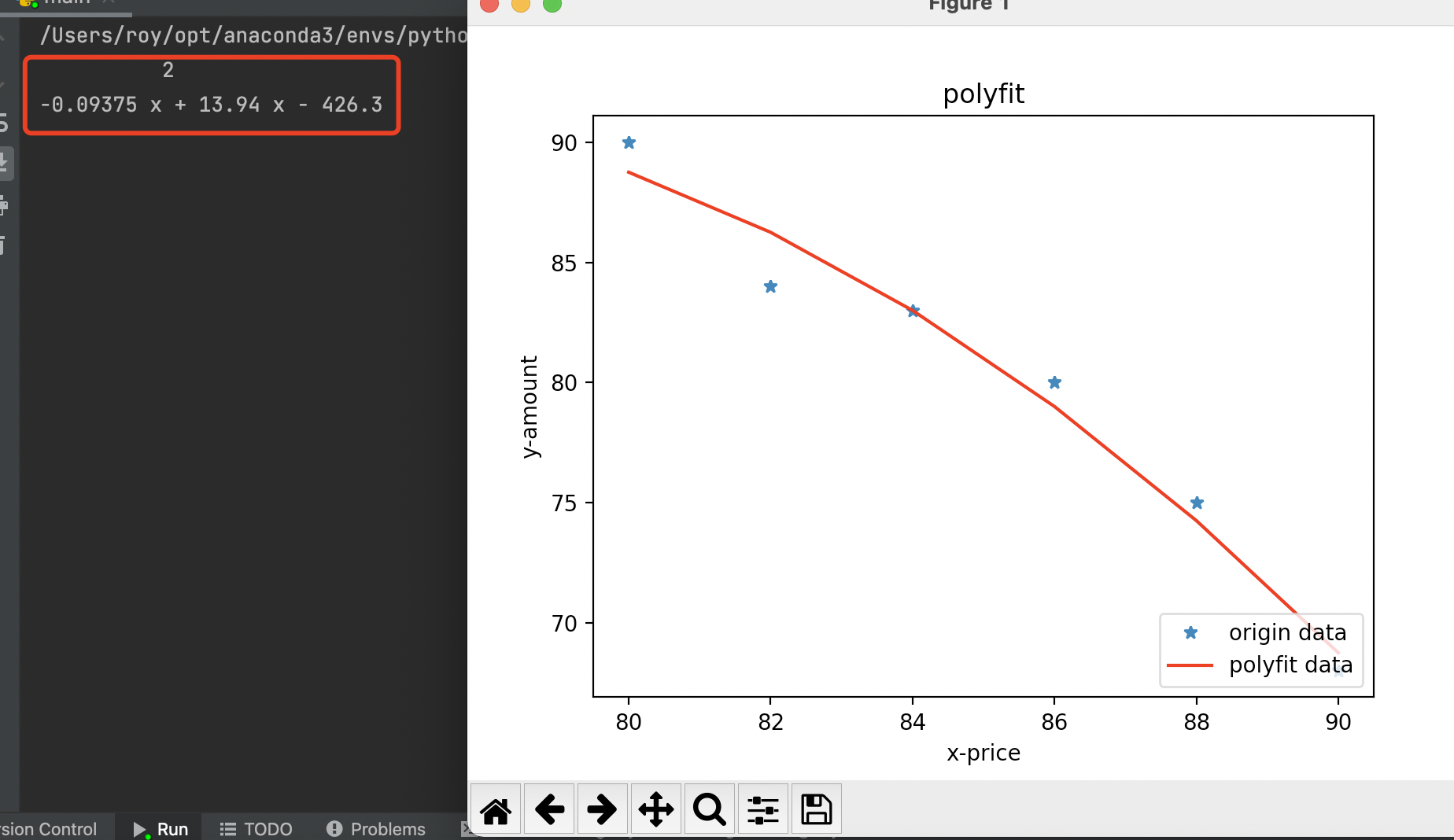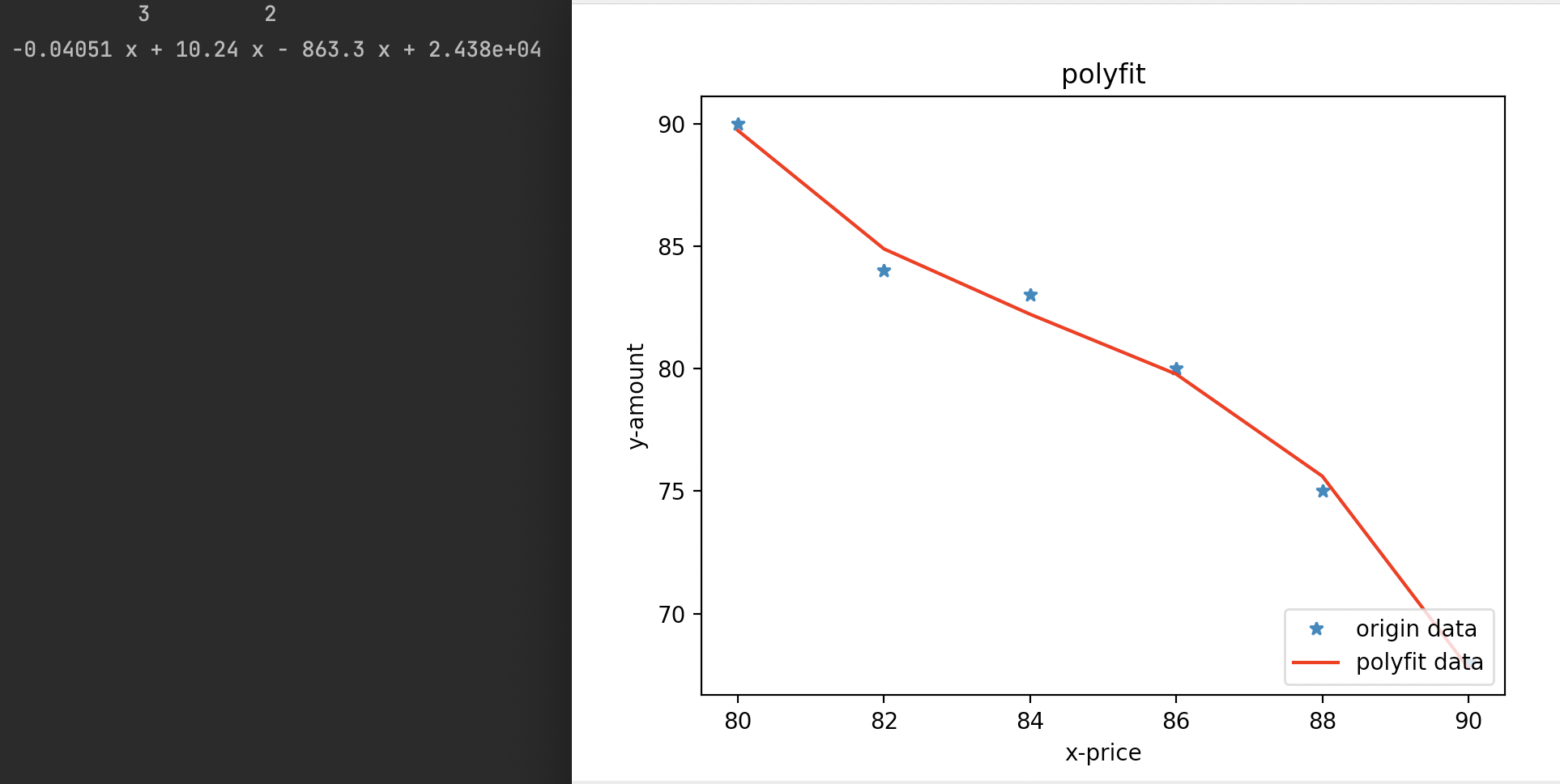# 一、背景描述# 二、前期准备

## 最小二乘法

(关于最小二乘法的原理大家可以自行了解,甚至可以让你重新感知到数学逻辑之美)

## Python类库

### NumPy

NumPy 是一个 Python 包。 它代表 “Numeric Python”。 它是一个由多维数组对象和用于处理数组的例程集合组成的库。支持大量的维度数组与矩阵运算，此外也针对数组运算提供大量的数学函数库。

### Matplotlib

Matplotlib 是 Python 的绘图库。 它可与 NumPy 一起使用，提供了一种有效的 MatLab 开源替代方案。

# 三、代码实现

``````import matplotlib.pyplot as plt
import numpy as np

# 准备数据,将(x,y)坐标点进行输入
x = np.arange(80, 92, 2)  # x = 80,82,84,86,88,90
y = np.array([90, 84, 83, 80, 75, 68])
# 使用polyfit方法来拟合,并选择多项式,这里先使用2次方程
z1 = np.polyfit(x, y, 2)
# 使用poly1d方法获得多项式系数,按照阶数由高到低排列
p1 = np.poly1d(z1)
# 在屏幕上打印拟合多项式
print(p1)
# 求对应x的各项拟合函数值
fx = p1(x)
# 绘制坐标系散点数据及拟合曲线图
plot1 = plt.plot(x, y, '*', label='origin data')
plot2 = plt.plot(x, fx, 'r', label='polyfit data')
plt.xlabel('x-price')
plt.ylabel('y-amount')
plt.legend(loc=4)  # 指定legend的位置,类似象限的位置
plt.title('polyfit')
plt.show()
plt.savefig('polyfit.png')

````````````z1 = np.polyfit(x, y, 3)
``````———————————————————————————我是分割线————————————————————————–
to be a better me, talk is cheap show me the code